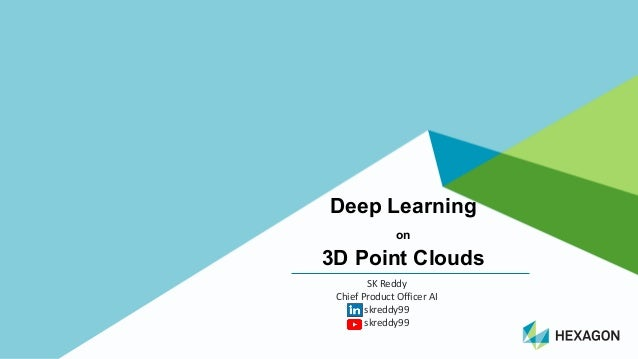Successfully reported this slideshow.Upcoming SlideShare
×

# 3D Point Cloud analysis using Deep Learning

208 views

Published on

Processing 3D images has many use cases. For example, to improve autonomous car driving, to enable digital conversions of old factory buildings, to enable augmented reality solutions for medical surgeries, etc. Also 3D images help in 3D modeling and safety evaluation of products.

3D image processing brings enormous benefits but also amplifies computing cost. The size of the point cloud, the number of points, sparse and irregular point cloud, and the adverse impact of the light reflections, (partial) occlusions, etc., make it difficult for engineers to process point clouds.

Moving from using hand crafted features to using deep learning techniques to semantically segment the images, to classify objects, to detect objects, to detect actions in 3D videos, etc., we have come a long way in 3D image processing.

3D Point Cloud image processing is increasingly used to solve Industry 4.0 use cases to help architects, builders and product managers. I will share some of the innovations that are helping the progress of 3D point cloud processing. I will share the practical implementation issues we faced while developing deep learning models to make sense of 3D Point Clouds.

Attendees: Beginners and Intermediate skilled in Image Processing and 3D Point Clouds

Profile of the speaker:

SK Reddy is the Chief Product Officer AI in Hexagon (www.hexagon.com). He is an AI and ML expert and a successful twice startup entrepreneur. He is an AI startup advisor too. Also he is a frequent speaker in conferences and is an AI blogger.

Published in: Technology
• Full Name
Comment goes here.

Are you sure you want to Yes No• Be the first to comment

• Be the first to like this

### 3D Point Cloud analysis using Deep Learning

1. 1. Deep\$Learning\$ on 3D\$Point\$Clouds SK#Reddy# Chief#Product#Officer#AI skreddy99 skreddy99
2. 2. Confidential2 https://arxiv.org/pdf/1808.01462.pdf
3. 3. Confidential3 https://arxiv.org/pdf/1808.01462.pdf
4. 4. Confidential4 O"CNN%&2017 https://arxiv.org/pdf/1712.01537.pdf Left:=the=original=3D=shape.=Middle:=the=voxelized 3D= shape.=Right:=the=octree=representation=with=normals sampled=at=the=finest=leaf=octants 2D=quadtree=illustration=of=octree=structure
5. 5. Confidential5 OctNet&'2017 https://arxiv.org/pdf/1611.05009.pdf Network=Architecture=Semantic=3D=Point=Cloud= Labeling Hybrid=GridKOctree=Data=Structure.=This=example=illustrates=a= hybrid=gridKoctree=consisting=of=8=shallow=octrees=indicated=by= different=colors.=Using=2=shallow=octrees=in=each=dimension= with=a=maximum=depth=of=3=leads=to=a=total=resolution=of======== voxels Bit=Representation.=Shallow=octrees=can=be=efficiently=encoded= using=bitKstrings.=Here,=the=bitKstring=1=01010000=00000000= 01010000=00000000=01010000=0...=defines=the=octree=in=(a).=The= corresponding=tree=is=shown=in=(b).=The=color=of=the=voxels= corresponds=to=the=split=level
6. 6. Confidential6 PointNet()2017 https://arxiv.org/pdf/1612.00593.pdf
7. 7. Confidential7 Deep\$Kd'Network.\$2017 https://arxiv.org/pdf/1704.01222.pdf A;kd=tree;built;on;the;point;cloud;of;eight;points;(left),; and;the;associated;Kd=network;built;for;classification; (right) The;architecture;for;parts;segmentation;(individual;point;classification); for;the;point;cloud
8. 8. Confidential8 PointNet++)*2017 https://arxiv.org/pdf/1706.02413.pdf (a) MultiAscaleCgroupingC(MSG)FC (b) MultiresolutionCgroupingC(MRG) MNISTCdigitCclassification
9. 9. Confidential9 SPLATNet)*2018* https://arxiv.org/pdf/1802.08275.pdf Bilateral*Convolution*Layer Left:=splatting=the=input=points=(orange)=onto= the=lattice=corners=(black)D=Middle:=The=extent=of=a=filter=on=the=lattice=with=a=s===2= neighborhood=(white=circles),=for=reference=we=show=a=Gaussian=filter,=with=its= values=color=coded.=The=general=case=has=a=free=scalar/vector=parameter=per= circle.=Right:=The=result=of=the=convolution=at=the=lattice=corners=(black)=is=projected= back=to=the=output=points=(blue).=
10. 10. Confidential10 MRTNet'(2018 https://arxiv.org/pdf/1807.03520.pdf
11. 11. Confidential11 SqueezeSeg:-CNNs-with-Recurrent-CRF-for-Real7Time-Road7 Object-Segmentation-from-3D-LiDAR-Point-CloudD-2017 https://arxiv.org/pdf/1710.07368.pdf Conditional-Random-Field-(CRF)-as-an-RNN-layer
12. 12. Confidential12 PointSeg-.Sep.2018 https://arxiv.org/pdf/1807.06288.pdf The.pipeline.of.PointSeg..Raw.LiDAR.point.cloud.is.projected.to.a.multichannel.range. image..After.the.network.processing,.the.predicted.pointKwise.label.will.be.projected.back. to.the.original.space. Source.code:.https://github.com/ywangeq/PointSeg
13. 13. Confidential13 LMNet:.Real0time.Multiclass.Object.Detection.on.CPU.using.3D.LiDAR?.2018 https://arxiv.org/pdf/1805.04902.pdf IMPLEMENTED.DILATED.LAYERS
14. 14. Confidential14 DeLS%3D:.Deep.Localization.and. Segmentation.with.a.3D.Semantic.Map;.2018 https://arxiv.org/pdf/1805.04949.pdf
15. 15. Confidential15 Spherical CNN for/3D/Point/Clouds5/2018 https://arxiv.org/pdf/1805.07872.pdf Classification/performance/on/ModelNetszz
16. 16. Confidential16 https://arxiv.org/pdf/1808.06840.pdf Example(result(of(our(FCPN(on(semantic(voxel(labeling(and(captioning(on(an(Tango(3D( reconstruction(/(point(cloud:((a)(3D(reconstruction((not(used),((b)(Input(point(cloud((c)(Output( semantic(voxel(prediction.( Visualization(of(the(semantic(segmentation(on((a)(a(depth(image,((b)(a(2.4m×2.4m×2.4m(partial( reconstruction((c)(an(entire(reconstruction(of(a(hotel(suite.(Please(note(that(each(of(these(outputs( are(predicted(in(a(single(shot(from(the(same(network(trained(with(2.4m(× 2.4m(× 2.4m(volumes( Fully%Convolutional-Point-Network-Architecture Aug<2018< (Source<code:<Not<yetC<https://github.com/drethage/fullyFconvolutionalFpointFnetwork)
17. 17. Confidential17 Data\$sets • ScanNet:2Indoor2Scenes • ShapeNet:23D2shapes • Princeton2ModelNet • KITTI2(Code2available2for2some) • Pascal3D+2(….in2the2wild) • Pascal2VOC • SUNCG2(room2models) • SceneNet (RGBMD2room2models) • CV2Lab2datasets2with2Code2 (http://cvgl.stanford.edu/resources.html) • Collective2Activity2Dataset2(old) • Monocular2Multiview2Object2Tracking2with2 3D2Aspect2Parts2(???) • Stanford22DM3DMSemantics2Dataset2(2DM 3DMS)2(**) • Stanford2Drone2Dataset • ObjectNet3D List\$of\$datasets: 1.2http://clickdamage.com/sourcecode/cv_datasets.php Other\$Data\$sets • TOSCA2(nonMrigid2dataset) • FAUST2(highMres2human2scans) • SUN3D • NYC3DCars • LabelMe3D2(old2dataset) • CVLab (MultiMview2car2dataset) • MIT2Street2Scenes • Daimler2Pedestrian2Datasets • Caltech2Pedestrian2Detection2Benchmark • Robust2MultiMPerson2Tracking2from2Mobile2Platforms • FlyingThings3D2(Synthetic) • BU4DFE23D2
18. 18. Confidential18 PVNet:"A"Joint"Network"of"Point"Cloud"and"Multi6View"for" 3D"Shape"Recognition"(Aug"2018)
19. 19. Confidential19 https://arxiv.org/pdf/1806.01411.pdf FlowNet3D:;Scene;Flow;in;3D;PCs(June;2018);
20. 20. Confidential20 YOLO3D Aug02018 Sample0of0the0output0shown0in03D0and0projected0on0the0top0view0map0
21. 21. Confidential21 PointFlowNet 3D/Scene/Flow/Estimation/from/PCs/(Sep/2018) https://arxiv.org/pdf/1806.02170.pdf
22. 22. Confidential22 Point&Cloud&Library Modules
23. 23. Confidential23 https://github.com/kzampog/cilantro; Cilantro library;for;point;cloud;data;processing; Bundled;with:; • nanoflann (for;fast;kdAtree;queries); • Spectra;(ARPACKAinspired;library;for;large; scale;eigen;decompositions; • Qhull (for;convex;hull;and;halfA space; intersection;computations); • tinyply (for;PLY;format;geometry;I/O); • External;dependencies:; • Eigen;(linear;algebra;library); • Pangolin;(lightweight;OpenGL;viewport; manager;and;video;I/O;abstraction;library)
24. 24. Confidential24 Thank&you SK&Reddy Chief&Product&Officer&AI skreddy99 skreddy99